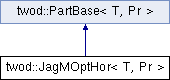# twod::JagMOptHor< T, Pr > Class Template Reference

An optimal m-way jagged algorithm based on dynamic programming. More...

#include <twod/jagged_dp.hpp>

Inheritance diagram for twod::JagMOptHor< T, Pr >:## Detailed Description

### template<typename T, typename Pr> class twod::JagMOptHor< T, Pr >

An optimal m-way jagged algorithm based on dynamic programming.

It takes the first dimension as the main dimension. Despite it is well optimized it is still fairly slow. It is based on the following dynamic programming formulation:.

Parameters:
 T type of the load Pr type of the prefix sum array

List of all members.

## Public Member Functions

JagMOptHor ()
virtual T part (int procCount, const Pr &prefixSumArray, util::RectList< T, Pr > &parts)
Applies a 2d partitioning algorithm to a given 2d prefixSumArray .
virtual ~JagMOptHor ()

## Static Private Member Functions

static T jagged_dp_internal (int procCount, const Pr &prefixSumArray, util::RectList< T, Pr > &parts)

## Constructor & Destructor Documentation

template<typename T, typename Pr>
 twod::JagMOptHor< T, Pr >::JagMOptHor ( )  [inline]
template<typename T, typename Pr>
 virtual twod::JagMOptHor< T, Pr >::~JagMOptHor ( )  [inline, virtual]

## Member Function Documentation

template<typename T , typename Pr >
 T twod::JagMOptHor< T, Pr >::jagged_dp_internal ( int procCount, const Pr & prefixSumArray, util::RectList< T, Pr > & parts )  [static, private]

build the rectangles of one stripe of the solution.

adds the solution of an optimal partition of the stripe [x:n] on m processors to parts.

Parameters:
 x first included column in the stripe n last included column in the stripe m number of processors to use in that stripe parts set of rectangle the solution will be added to.

provide the optimal (load) partition of one stripe.

More precisely the [x:n] stripe on m processors.

Parameters:
 x the first included column. n the last included column. m how many processors to use.
Returns:
the optimal value of the 1d partitioning of that stripe.

provide a lower bound on the optimal (load) partition of one stripe.

More precisely the [x:n] stripe on m processors.

Parameters:
 x the first included column. n the last included column. m how many processors to use.
Returns:
a lower bound on the optimal value of the 1d partitioning of that stripe.

return a lower bound on the best partitioning of [1:n] on m processors using m-way jagged partitioning

return an upper bound bound on the best partitioning of [1:n] on m processors

provides an upper bound of the load of a stripe for a given number of processors.

More precisely of [x:n] jagged on m processors.

Parameters:
 x is the first column included n is the last column included m is the number of processors considers
Returns:
returns an upper bound on the value of the partition of the stripe.

fill one element of the DP array by computing its value. Notice that it calls compute which will fills other value in the array if required.

naively fill the whole DP array. Very slow, should probably not be used.

backtrack in the DP array and add the rectangle of the optimal solution to parts

Parameters:
 parts is added the rectangle of the built solution

compute the value of the optimal partition

changes the upper bound on the best known jagged partition

Parameters:
 b the new upper bound. Should be given by a valid m-way jagged partition

template<typename T , typename Pr >
 T twod::JagMOptHor< T, Pr >::part ( int procCount, const Pr & prefixSumArray, util::RectList< T, Pr > & parts )  [virtual]

Applies a 2d partitioning algorithm to a given 2d prefixSumArray .

Parameters:
 [in] procCount is the number of processors [in] prefixSumArray first column and first row consists of zero only. But the borders of rectangles in rect_list never touch this area (index 0 in row or column) [out] parts must be empty before calling this function. It will contain the partition after the function return
Returns: